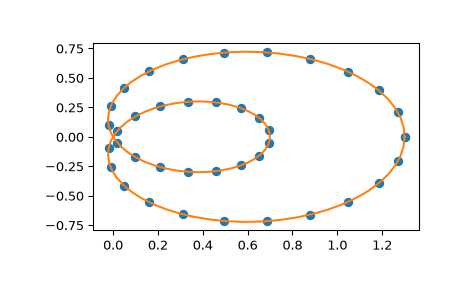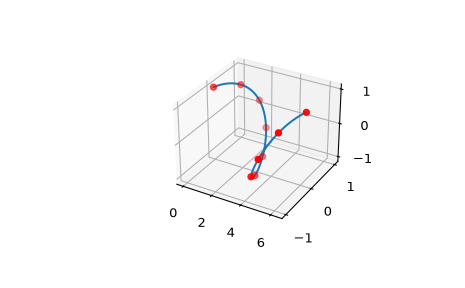# scipy.interpolate.make_interp_spline¶

scipy.interpolate.make_interp_spline(x, y, k=3, t=None, bc_type=None, axis=0, check_finite=True)[source]

Compute the (coefficients of) interpolating B-spline.

Parameters
xarray_like, shape (n,)

Abscissas.

yarray_like, shape (n, …)

Ordinates.

kint, optional

B-spline degree. Default is cubic, k=3.

tarray_like, shape (nt + k + 1,), optional.

Knots. The number of knots needs to agree with the number of datapoints and the number of derivatives at the edges. Specifically, `nt - n` must equal `len(deriv_l) + len(deriv_r)`.

bc_type2-tuple or None

Boundary conditions. Default is None, which means choosing the boundary conditions automatically. Otherwise, it must be a length-two tuple where the first element sets the boundary conditions at `x` and the second element sets the boundary conditions at `x[-1]`. Each of these must be an iterable of pairs `(order, value)` which gives the values of derivatives of specified orders at the given edge of the interpolation interval. Alternatively, the following string aliases are recognized:

• `"clamped"`: The first derivatives at the ends are zero. This is

equivalent to `bc_type=([(1, 0.0)], [(1, 0.0)])`.

• `"natural"`: The second derivatives at ends are zero. This is equivalent to `bc_type=([(2, 0.0)], [(2, 0.0)])`.

• `"not-a-knot"` (default): The first and second segments are the same polynomial. This is equivalent to having `bc_type=None`.

• `"periodic"`: The values and the first `k-1` derivatives at the ends are equivalent.

axisint, optional

Interpolation axis. Default is 0.

check_finitebool, optional

Whether to check that the input arrays contain only finite numbers. Disabling may give a performance gain, but may result in problems (crashes, non-termination) if the inputs do contain infinities or NaNs. Default is True.

Returns
ba BSpline object of the degree `k` and with knots `t`.

`BSpline`

base class representing the B-spline objects

`CubicSpline`

a cubic spline in the polynomial basis

`make_lsq_spline`

a similar factory function for spline fitting

`UnivariateSpline`

a wrapper over FITPACK spline fitting routines

`splrep`

a wrapper over FITPACK spline fitting routines

Examples

Use cubic interpolation on Chebyshev nodes:

```>>> def cheb_nodes(N):
...     jj = 2.*np.arange(N) + 1
...     x = np.cos(np.pi * jj / 2 / N)[::-1]
...     return x
```
```>>> x = cheb_nodes(20)
>>> y = np.sqrt(1 - x**2)
```
```>>> from scipy.interpolate import BSpline, make_interp_spline
>>> b = make_interp_spline(x, y)
>>> np.allclose(b(x), y)
True
```

Note that the default is a cubic spline with a not-a-knot boundary condition

```>>> b.k
3
```

Here we use a ‘natural’ spline, with zero 2nd derivatives at edges:

```>>> l, r = [(2, 0.0)], [(2, 0.0)]
>>> b_n = make_interp_spline(x, y, bc_type=(l, r))  # or, bc_type="natural"
>>> np.allclose(b_n(x), y)
True
>>> x0, x1 = x, x[-1]
>>> np.allclose([b_n(x0, 2), b_n(x1, 2)], [0, 0])
True
```

Interpolation of parametric curves is also supported. As an example, we compute a discretization of a snail curve in polar coordinates

```>>> phi = np.linspace(0, 2.*np.pi, 40)
>>> r = 0.3 + np.cos(phi)
>>> x, y = r*np.cos(phi), r*np.sin(phi)  # convert to Cartesian coordinates
```

Build an interpolating curve, parameterizing it by the angle

```>>> from scipy.interpolate import make_interp_spline
>>> spl = make_interp_spline(phi, np.c_[x, y])
```

Evaluate the interpolant on a finer grid (note that we transpose the result to unpack it into a pair of x- and y-arrays)

```>>> phi_new = np.linspace(0, 2.*np.pi, 100)
>>> x_new, y_new = spl(phi_new).T
```

Plot the result

```>>> import matplotlib.pyplot as plt
>>> plt.plot(x, y, 'o')
>>> plt.plot(x_new, y_new, '-')
>>> plt.show()
```Build a B-spline curve with 2 dimensional y

```>>> x = np.linspace(0, 2*np.pi, 10)
>>> y = np.array([np.sin(x), np.cos(x)])
```

Periodic condition is satisfied because y coordinates of points on the ends are equivalent

```>>> ax = plt.axes(projection='3d')
>>> xx = np.linspace(0, 2*np.pi, 100)
>>> bspl = make_interp_spline(x, y, k=5, bc_type='periodic', axis=1)
>>> ax.plot3D(xx, *bspl(xx))
>>> ax.scatter3D(x, *y, color='red')
>>> plt.show()
```